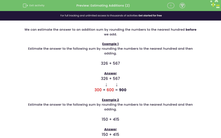# Estimate Additions by Rounding to the Nearest 100

In this worksheet, students will estimate the answer to addition sums by rounding two numbers to the nearest hundred and then adding.Key stage:  KS 2

Curriculum topic:   Number: Addition and Subtraction

Curriculum subtopic:   Estimate and Check Answers

Popular topics:   Estimation worksheets

Difficulty level:#### Worksheet Overview

We can estimate the answer to an addition sum by rounding the numbers to the nearest hundred before we add.

Remember that if a number ends in 50 or above, it rounds up to the next hundred.

If a number ends in 49 or anything below, it rounds down to the previous hundred.

Example 1

Estimate the answer to the following sum by rounding the numbers to the nearest hundred and then adding.

326 + 567

326 + 567

↓        ↓

300 + 600 = 900

Example 2

Estimate the answer to the following sum by rounding the numbers to the nearest hundred and then adding.

150 + 415

150 + 415

↓        ↓

200 + 400 = 600

Remember that 150 rounds up to 200, 350 rounds up to 400, 250 rounds up to 300 etc.

Are you ready to try some questions now?### What is EdPlace?

We're your National Curriculum aligned online education content provider helping each child succeed in English, maths and science from year 1 to GCSE. With an EdPlace account you’ll be able to track and measure progress, helping each child achieve their best. We build confidence and attainment by personalising each child’s learning at a level that suits them.

Get started